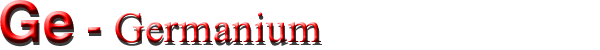Optical properties

 Infrared refractive index n 4.00 Radiative recombination coefficient 6.4·10-14 cm3/s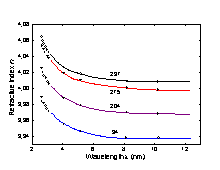Refractive index n versus wavelength at four different temperatures. (Icenogle et al. ).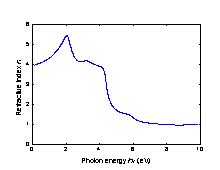Refractive index n versus photon energy. T = 300 K. (Philipp and Taft ).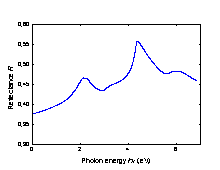Reflectance versus photon energy. T = 300 K. (Cordona et al. ).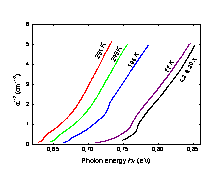Low-level absorption spectrum of high purity Ge at various temperatures. (Macfarlane et al. ).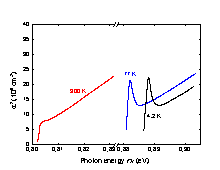The absorption edge at three different temperatures (Seysyan et al. ).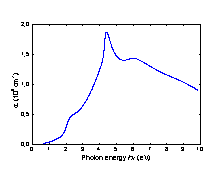The absorption coefficient versus photon energy. T = 300 K. (Phillipp and Taft ).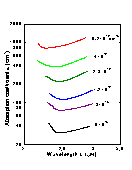The absorption coefficient at different donor (As) concentrations. T = 300 K (Pankove and Aigrain ).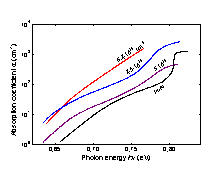Intrinsic absorption edges at different donor (As) concentrations at 300 K obtained after substracting the free carrier absorption from the measured values. (Pankove and Aigrain ).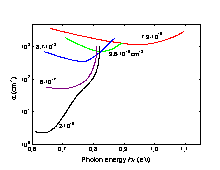T = 293 K. The absorption coefficient at different acceptor (Ga) concentrations. (Bagaev et al. ).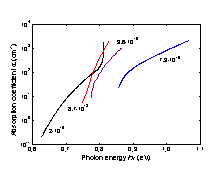Intrinsic absorption edge at different doping (Ga) level T = 293 K. (Bagaev et al. ).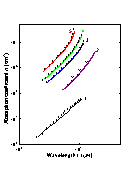Free carrier absorption versus wavelength at different doping levels. n-Ge. T = 300 K. Conduction electron concentration: no(cm-1): 1. 8.0·1017; 2. 4.8·1018; 3. 1.35·1019; 4. 1.8·1019; 5. 3.6·1019. (Fistul )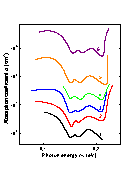Free carrier absorption versus photon energy at different doping levels. p-Ge. T = 300 K. Free hole concentration: po(cm-3): 1. 7.3·1015; 2. 1.6·1016; 3. 6.0·1016; 4. 1.9·1017; 5. 1.2·1018; 6. 1.0·1019. (Ukhanov  and Vasilyeva et al. ).

Two-photon absorption coefficient β

 λ τp, ns β×1011, m W-1 Ref. 2.36 30 50±0.3 (Zubov et al. ). 2.6-3.1 100 2500 (?) (Wenzel et al. ). 480 340 (Gibson et al. ).